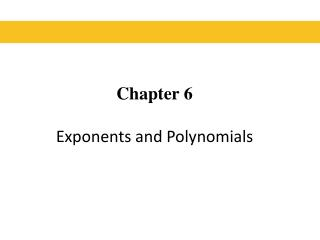DownloadDownload PresentationChapter 6 Exponents and Polynomials

# Chapter 6 Exponents and Polynomials

Télécharger la présentation## Chapter 6 Exponents and Polynomials

- - - - - - - - - - - - - - - - - - - - - - - - - - - E N D - - - - - - - - - - - - - - - - - - - - - - - - - - -
##### Presentation Transcript

1. Chapter 6Exponents and Polynomials

2. §6.1 Adding and Subtracting Polynomials

3. Polynomials A polynomial is a single term or the sum of two or more terms containing variables with whole number exponents. Consider the polynomial: This polynomial contains four terms. It is customary to write the terms in order of descending powers of the variable. This is the standard form of a polynomial. Here are two other polynomials which are written in standard form. Blitzer, Introductory Algebra, 5e – Slide #3 Section 6.1

4. Polynomials The degree of a polynomial is the greatest degree of any term of the polynomial. The degree of a term is (n +m) and the coefficient of the term is a. If there is exactly one term of greatest degree, it is called the leading term. It’ s coefficient is called the leading coefficient. Consider the polynomial: 3 is the leading coefficient. The degree is 4. Blitzer, Introductory Algebra, 5e – Slide #4 Section 6.1

5. Polynomials The Degree of axn • If a ≠ 0, the degree of axn is n. The degree of a nonzero constant term is 0. The constant 0 has no defined degree. Degree of nonzero constant: 0 Degree 2 Degree 1 Degree 3 Blitzer, Introductory Algebra, 5e – Slide #5 Section 6.1

6. Degree of a Polynomial • The degree of a polynomial is the degree of its highest order term. • Example: Degree 3 Polynomial: Degree 4 Polynomial: Blitzer, Introductory Algebra, 5e – Slide #6 Section 6.1

7. Special Polynomials • Monomial: A polynomial with one term. • Binomial: A polynomial with two terms. • Trinomial: A polynomial with three terms. Example: This is a 4th degree trinomial. Blitzer, Introductory Algebra, 5e – Slide #7 Section 6.1

8. Polynomials Blitzer, Introductory Algebra, 5e – Slide #8 Section 6.1

9. Adding Polynomials • Polynomials are added by combining like terms. • Like terms are terms containing exactly the same variables to the same powers. • Example: These like terms both contain x to the second power. Add the coefficients and keep the same variable factor, x2 Blitzer, Introductory Algebra, 5e – Slide #9 Section 6.1

10. Adding Polynomials EXAMPLE Group like terms. Combine like terms. Blitzer, Introductory Algebra, 5e – Slide #10 Section 6.1

11. Adding Polynomials EXAMPLE Add: SOLUTION Remove parentheses Rearrange terms so that like terms are adjacent Combine like terms Blitzer, Introductory Algebra, 5e – Slide #11 Section 6.1

12. Subtracting Polynomials EXAMPLE Add the opposite of the polynomial being subtracted. Group like terms. Combine like terms. Blitzer, Introductory Algebra, 5e – Slide #12 Section 6.1

13. Subtracting Polynomials EXAMPLE Subtract SOLUTION Change subtraction to addition and change the sign of every term of the polynomial in parentheses. Rearrange terms Combine like terms Blitzer, Introductory Algebra, 5e – Slide #13 Section 6.1

14. Polynomial Functions is an example of a polynomial function. In a polynomial function, the expression that defines the function is a polynomial. How do you evaluate a polynomial function? Use Substitution. Blitzer, Introductory Algebra, 5e – Slide #14 Section 6.1

15. Graphs of Polynomial Functions Polynomial functions of degree 2 or higher have graphs that are smooth and continuous. By smooth, we mean that the graph contains only rounded corners with no sharp corners. By continuous, we mean that the graph has no breaks and can be drawn without lifting the pencil from the page. Blitzer, Introductory Algebra, 5e – Slide #15 Section 6.1

16. Graphs of Polynomials EXAMPLE Smooth rounded curve The graph below does not represent a polynomial function. Although it has a couple of smooth, rounded corners, it also has a sharp corner and a break in the graph. Either one of these last two features disqualifies it from being a polynomial function. Sharp Corner Discontinuous break Smooth rounded curve Blitzer, Introductory Algebra, 5e – Slide #16 Section 6.1